Warning: Parameter 1 to wp_default_styles() expected to be a reference, value given in /home/public/wp-includes/plugin.php on line 600
Comments for BikeSoccer http://www.bikesoccer.com Sat, 05 May 2012 09:45:46 +0000 hourly 1 https://wordpress.org/?v=4.6.1 Comment on Is massively collaborative mathematical physics possible? by Clark Alexander http://www.bikesoccer.com/2012/04/07/is-massively-collaborative-mathematical-physics-possible/#comment-14 Sat, 05 May 2012 09:45:46 +0000 http://www.bikesoccer.com/?p=67#comment-14 If we simply set up the differential equation as a power series we arrive at the recurrence relationSadly this is a 6th order equation rather than a second order equation.
So it seems we need to know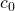through]]>
Comment on Is massively collaborative mathematical physics possible? by Clark Alexander http://www.bikesoccer.com/2012/04/07/is-massively-collaborative-mathematical-physics-possible/#comment-13 Sun, 15 Apr 2012 17:01:57 +0000 http://www.bikesoccer.com/?p=67#comment-13 Sorry for the three posts, I obviously made a few typing mistakes the first two times.

]]>
Comment on Is massively collaborative mathematical physics possible? by Clark Alexander http://www.bikesoccer.com/2012/04/07/is-massively-collaborative-mathematical-physics-possible/#comment-12 Sun, 15 Apr 2012 11:59:54 +0000 http://www.bikesoccer.com/?p=67#comment-12 For the ground state of the the quartic oscillator, it appears we can make some rapid progress…

When calculating commutators that give ground state energies, the only surviving terms are of the form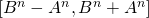These, of course, yield higher order terms, but the constant term given is simply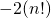.

When looking at the second order, we also know that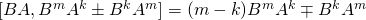So our commutators will carry the extra terms…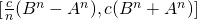times an additional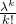.

So it looks like the quartic ground state will be the summation of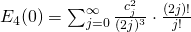or something like this.

The problem is how fast the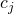will diverge. It appears that this will be difficult to resume if thediverge at all.
Again, according to Bender and Wu, the overall terms grows asymptotically like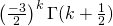which means thatappear to diverge but at some algebraic rate, perhaps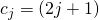.
Not sure…

]]>
Comment on Is massively collaborative mathematical physics possible? by Clark Alexander http://www.bikesoccer.com/2012/04/07/is-massively-collaborative-mathematical-physics-possible/#comment-11 Sun, 15 Apr 2012 11:56:52 +0000 http://www.bikesoccer.com/?p=67#comment-11 For the ground state of the the quartic oscillator, it appears we can make some rapid progress…

When calculating commutators that give ground state energies, the only surviving terms are of the formThese, of course, yield higher order terms, but the constant term given is simply.

When looking at the second order, we also know thatSo our commutators will carry the extra terms…times an additional.

So it looks like the quartic ground state will be the summation ofor something like this.

The problem is how fast thewill diverge. It appears that this is in good standing for resummability if thegrow larger than this.
Again, according to Bender and Wu, the overall terms grows asymptotically likewhich means thatmight diverge incredibly fast.

]]>
Comment on Is massively collaborative mathematical physics possible? by Clark Alexander http://www.bikesoccer.com/2012/04/07/is-massively-collaborative-mathematical-physics-possible/#comment-10 Sun, 15 Apr 2012 11:55:51 +0000 http://www.bikesoccer.com/?p=67#comment-10 For the ground state of the the quartic oscillator, it appears we can make some rapid progress…

When calculating commutators that give ground state energies, the only surviving terms are of the formThese, of course, yield higher order terms, but the constant term given is simply.

When looking at the second order, we also know thatSo our commutators will carry the extra terms…times an additional.

So it looks like the quartic ground state will be the summation ofor something like this.

The problem is how fast thewill diverge. It appears that this is in good standing for resummability if thegrow larger than this.
Again, according to Bender and Wu, the overall terms grows asymptotically likewhich means thatmight diverge incredibly fast.

]]>
Comment on Is massively collaborative mathematical physics possible? by Clark Alexander http://www.bikesoccer.com/2012/04/07/is-massively-collaborative-mathematical-physics-possible/#comment-9 Sat, 14 Apr 2012 18:10:58 +0000 http://www.bikesoccer.com/?p=67#comment-9 Here’s a guess at the energy levels for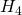.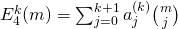In this case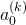will be our ground state correction for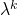.
One thing we can see easily is that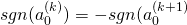.
In fact we know from Bender and Wu (ca.1963).

]]>
Comment on Is massively collaborative mathematical physics possible? by Clark Alexander http://www.bikesoccer.com/2012/04/07/is-massively-collaborative-mathematical-physics-possible/#comment-8 Sat, 14 Apr 2012 18:04:55 +0000 http://www.bikesoccer.com/?p=67#comment-8 Now that I can write these things a little more nicely:

Let me write these three term energy values as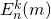where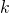corresponds to thecorrection,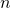corresponds to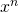the power of the overall perturbation, and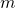corresponds to the energy level of the eigenstate.

So here we have:when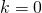and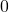otherwise.whenand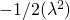whenotherwise.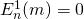ifis odd.when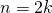.

Furthermore, we know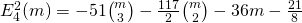.

The question becomes whether we can define some function (or sequence of functions) which may function as “differentials” so that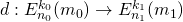.

]]>
Comment on Is massively collaborative mathematical physics possible? by Clark Alexander http://www.bikesoccer.com/2012/04/07/is-massively-collaborative-mathematical-physics-possible/#comment-7 Sat, 14 Apr 2012 11:29:22 +0000 http://www.bikesoccer.com/?p=67#comment-7 In the same way that we can view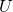andas differential operators in “standard coordinates” we can realize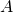andas “differential operators” ofandrespectively.meansand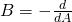.

]]>
Comment on Is massively collaborative mathematical physics possible? by Clark Alexander http://www.bikesoccer.com/2012/04/07/is-massively-collaborative-mathematical-physics-possible/#comment-6 Wed, 11 Apr 2012 22:19:19 +0000 http://www.bikesoccer.com/?p=67#comment-6 If we move the U operators over we have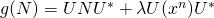In this case we may be able to convertfrom being in terms ofandto being in terms ofand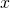. Luckily (let’s call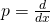for the moment)

We can mover the piece furthest to the right into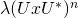But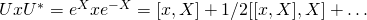and if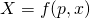then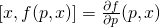which makes our commutators a little easier.

]]>
Comment on Is massively collaborative mathematical physics possible? by Clark Alexander http://www.bikesoccer.com/2012/04/07/is-massively-collaborative-mathematical-physics-possible/#comment-5 Tue, 10 Apr 2012 17:47:21 +0000 http://www.bikesoccer.com/?p=67#comment-5 Calling all the topologists out there:

Is there a way to setup a spectral sequence structure on the perturbed eigenvalues?

Consider that we need to compute these values…
1) lambda^k (in the power series)
2) x^n (the perturbation in the potential)
3) the m^th energy level for the x^n potential.

Therefore we’re looking for the terms E^k_{n,m}.

What’s known so far are
i) E^0_{n,m} all n,m
ii) E^1_{n,m} all n,m (solved by A.)
iii) E^k_{0,m} all k,m
iv) E^k_{1,m} all k,m
v) E^2_{4,m} all m (solved by A.)

What’s also known is that

E^k_{n,m} implies E^{k+1}_{n,0}
we get the next ground state if we know the entire previous solution.

Can we set up some sort of differential structure here?
Perhaps also we won’t have a d^2=0 but perhaps d^j = 0 for some other j…

Maybe
d^j : E^k_{n,m} rightarrow E^{k+1}_{n,m-j} or something like this…

]]>